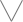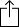C♭
D♭
E♭
F♭
G♭
A♭
B♭
Chord
Scale
Custom
3nps
CAGED
None
Notes
Degrees
Intervals
None

1
2
3
4
5
1
2
3
4
5
6
7
8
9
10
11
12
13
14
15
16
17
18
19
20
21
22
23
24
E
B
G
D
A
E
B
F
G
A
A
B
B
C
C
D
E
E
F
F
G
A
A
B
B
C
C
D
E
E
F
C
D
E
E
F
F
G
A
A
B
B
C
C
D
E
E
F
F
G
A
A
B
B
C
A
B
B
C
C
D
E
E
F
F
G
A
A
B
B
C
C
D
E
E
F
F
G
A
E
F
F
G
A
A
B
B
C
C
D
E
E
F
F
G
A
A
B
B
C
C
D
E
B
C
C
D
E
E
F
F
G
A
A
B
B
C
C
D
E
E
F
F
G
A
A
B
F
G
A
A
B
B
C
C
D
E
E
F
F
G
A
A
B
B
C
C
D
E
E
F
C
D
E
E
F
F
G
A
A
B
B
C
C
D
E
E
F
F
G
A
A
B
B
C

Fb Minor Pentatonic Scale for 7 String Guitar - CAGED Chart

Fb Minor Pentatonic Scale is a pentatonic scale. Pentatonic scales have 5 notes, "penta" comes from the greek word "Pentateuch" which means 5.

The Fb Minor Pentatonic Scale scale is derived from the Fb Minor scale by taking scale degree 1, 3, 4, 5 and 7 of the scale.

F
A
B
C
E
Fm3
W
W
m3
W
Play scaleand then